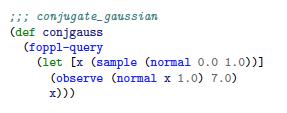# Pytorch graphs for inference

Hi,

I’ve been using Pytorch to create a Hamiltonian Monte Carlo (HMC) inference algorithm for directed graphical models. However, some of these graphical models can be quite large and all that is changing within the graph is the value of the latent variables, which have the flag requires_grad = True. As with each iteration we generate new proposals with the HMC algorithm which are then reassigned to the values of the latent variables, if they are accepted at the proposal step.
I’m currently using the torch.autograd.grad() function whenever I compute the gradients, as with backward, because it propagates all the way through the graph it can add additional things to the gradients of the variables. By the way, a digamma and beta function implementation in PyTorch would be super awesome for the inference community and the ability to do Choelsky decomposition with variable objects would also be awesome, as torch.potrf() only allows tensors.
The graphical model is contained within another module and it has to recreate the graph each time, despite the structure not changing and only the data of the latent variables variable object.
I love the dynamic graph structure of PyTorch, hence why I am not using TF, but is there a way to do this kind of computation more efficiently. I can provide code, if more details are needed.

• digamma and beta functions, noted.
• ability to do `torch.potrf` on variables - noted.

Will try to prioritize them for the next release.

I couldn’t quite get what you were talking about on the recreation of the graph, could you please elaborate?

1 Like

Hi,

Thanks for the response and sorry for the very long response, I hope that the problem will become clearer.
I shall also add that members of the group that I am currently working in with are creating distribution classes in PyTorch and we are more than happy to contribute to developing a scipy.stats style package in PyTorch, as it will not only be useful to us, but I imagine a lot of others too .

So for example, take a simple conjugate Gaussian model:Where the latent variable is x and the observed value is y.
When the above code is translated into python code, we get something like this:
*note VariableCast is just a function that we wrote that converts scalars, numpy arrays and tensors to Pytorch variables, with requires_grad= False. BaseClass is what all ‘programs’ (a graphical model) inherit, that contains a method called calc_grad(output, input), which basically just calls torch.autograd.grad(output=‘log of the joint of the graphical model’, input = ‘latent parameters’) and returns the gradients of the log joint w.r.t latent parameters:

``````class conjgauss(BaseProgram):
``````
``````         def __init__(self):
super().__init__()

def generate(self):
''' Generates the initial state and returns the samples and logjoint
Only called at the start of the HMC Loop '''

################## Start FOPPL input ##########
logp = [] # empty list to store logp's of each latent parameter
dim = 1
params = Variable(torch.FloatTensor(1,dim).zero_())
mean1 = VariableCast(0.0)
std1 = VariableCast(2.236)
normal_object = Normal(mean1, std1)
x = Variable(normal_object.sample().data, requires_grad = True)
params = x

std2    = VariableCast(1.4142)
y       = VariableCast(7.0)
p_y_g_x    = Normal(params[0,:], std2)

################# End FOPPL output ############
dim_values = params.size()
logp.append(normal_object.logpdf(params))
logp.append(p_y_g_x.logpdf(y))

# sum up all log probabilities
logp_x_y   = VariableCast(torch.zeros(1,1))
for logprob in logp:
logp_x_y = logp_x_y + logprob

'''This will be continually called within the leapfrog step
'''
logp = []  # empty list to store logp's of each latent parameter
assert (isinstance(values, Variable))
################## Start FOPPL input ##########
values = Variable(values.data, requires_grad = True) # This is our new 'x'
mean1 = VariableCast(0.0)
std1 = VariableCast(2.236)
normal_object = Normal(mean1, std1)

std2 = VariableCast(1.4142)
y      = VariableCast(7.0)
p_y_g_x    = Normal(values[0,:], std2)

logp.append(normal_object.logpdf(values[0,:]))
logp.append(p_y_g_x.logpdf(y))

################# End FOPPL output ############
logjoint = VariableCast(torch.zeros(1, 1))

for logprob in logp:
logjoint = logjoint + logprob